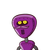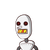# 6 pumps are required to fill a tank in 1hour 20 minutes how much long will it take to if only 5 pumps of same type are used​

6 pumps are required to fill a tank in 1hour 20 minutes how much long will it take to if only 5 pumps of same type are used​

### 2 thoughts on “6 pumps are required to fill a tank in 1hour 20 minutes how much long will it take to if only 5 pumps of same type are used​”

1.1 hr 36 minutes

Step-by-step explanation:

$$\left[\begin{array}{ccc}Pumps&Time\\6&80 mins\\5&x\end{array}\right]$$

Since more pumps = less time and less pumps = more time,

The values are indirectly proportionate.

x = $$\frac{6*80}{5}$$

x = 96 mins = 1 hr 36 minutes

I hope this helps you . . . please comment if further doubt.

2.Step-by-step explanation:

6 pumps can fill a tank in 1 + 1/3 = 4/3hrs

1 pump fill it in = 4/3 * 6 = 8 hrs

5 pump can fill in = 8*5 = 40 hrs.